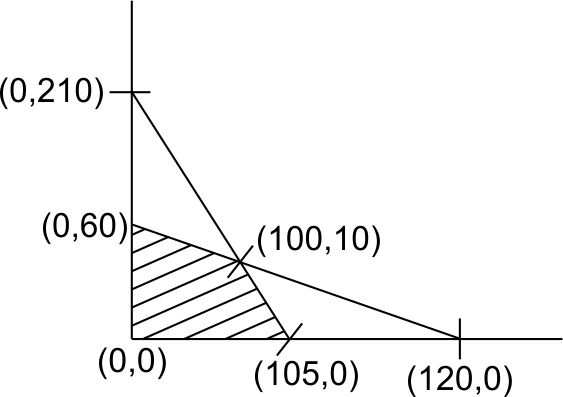# Consider the following linear programming problemMaximize 5x+6ySubject to, 1x+2y120all variables0which of the following points (x,y) is in feasible region? Option 1)Option 2)Option 3)Option 4) None of the above

As we learnt in

Decision Variables -

In Z = ax+by, x and y are decision variables.

-Z = 5x + 6y,Z = 360 (0, 60)

Z = 0 (0,0)

Z = 525 (105,0)

Z = 560 (100,10)

Maximise at (100, 10)

Option 1)incorrect

Option 2)incorrect

Option 3)incorrect

Option 4)

None of the above

correct

### Preparation Products

##### Knockout BITSAT 2021

It is an exhaustive preparation module made exclusively for cracking BITSAT..

₹ 4999/- ₹ 2999/-